5.1.6. Decay Rates and Spectrum

The part of the weak Hamiltonian responsible for the decay pn + e+ +e is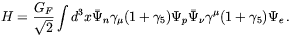(5.31)

For non-relativistic heavy particles the matrix element of this Hamiltonian between a proton with quantum numbers pz = 0 , m = mi, a neutron with momentum pn, a neutrino with momentum pand an electron in state m = mf and with pz,e is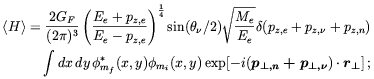(5.32)is the azimuthal angle of the neutrino. The integral in the above expression can be evaluated in a multipole expansion. Note that the natural extent of the integral in the transverse direction is 1 / sqrt[eB] whereas the neutron momenta are, from Eq. (5.27), of the order of sqrt[eB(0.12 + 0.04B14)]; thus setting the exponential term in this integral equal to one will yield a good estimate for the rate and spectrum of this decay. The positron spectrum is given by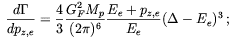(5.33)

where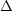is defined in Eq. (5.27). For>> Me the total rate is easily obtained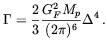(5.34)

For B = 5 × 1014 T, the lifetime is= 6 s.